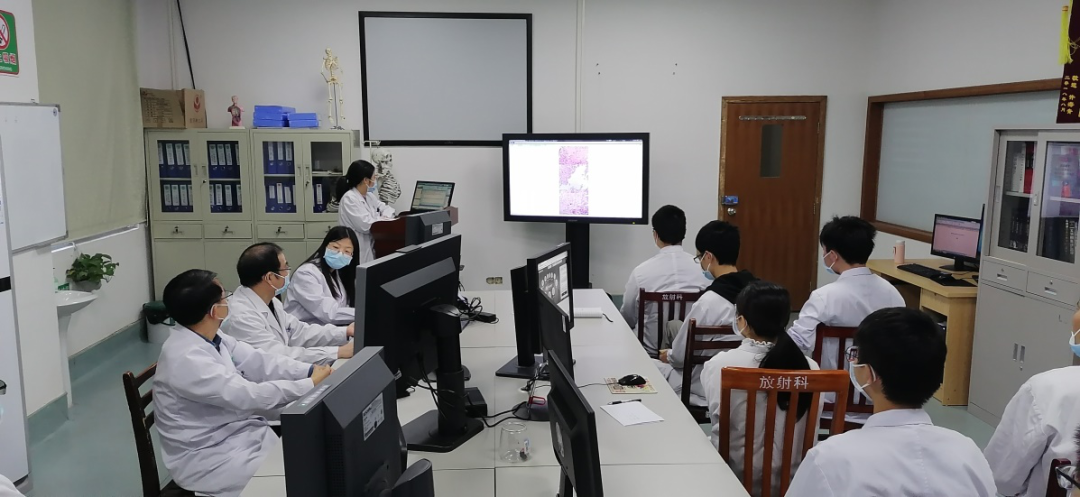### 今天是 var day = ""; var month = ""; var ampm = ""; var ampmhour = ""; var myweekday = ""; var year = ""; mydate = new Date(); myweekday = mydate.getDay(); mymonth = mydate.getMonth() + 1; myday = mydate.getDate(); myyear = mydate.getYear(); year = (myyear > 200) ? myyear : 1900 + myyear; if (myweekday == 0) weekday = " 星期日 "; else if (myweekday == 1) weekday = " 星期一 "; else if (myweekday == 2) weekday = " 星期二 "; else if (myweekday == 3) weekday = " 星期三 "; else if (myweekday == 4) weekday = " 星期四 "; else if (myweekday == 5) weekday = " 星期五 "; else if (myweekday == 6) weekday = " 星期六 "; document.write("" + year + "年" + mymonth + "月" + myday + "日 " + weekday + "");   67777.com祝您身体健康！家庭幸福！

67777.com网站地图手机版微信公众号办公系统图书馆

# 【我为群众办实事】CT/MRI预约等候时间再缩短——解决看病难又有新举措